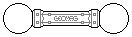Web textodigital.com
The rhombic hexecontahedron
The rhombic hexecontahedron is a solid made of 60 rhombuses which form 12 concave pentagonal stars. It can be easily thought of as a regular dodecahedron whose faces and edges have sunk in their middle, towards the center of the object: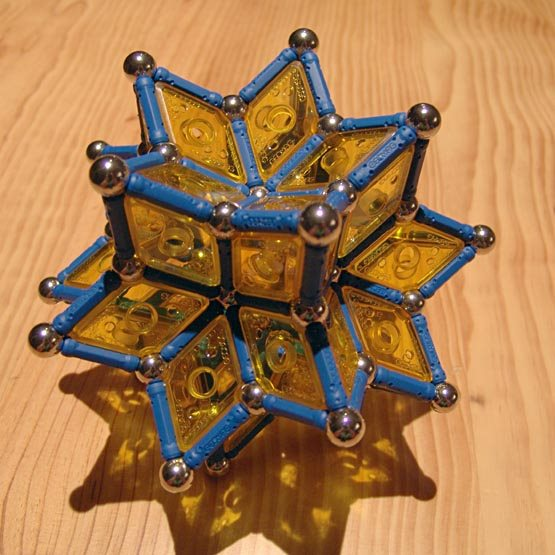Rhombic hexecontahedron to scale 1 242 pieces: 62 balls, 120 rods, 60 rhombuses (1.18 kg)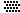Of a total of 62 vertices, the 12 concave ones in the middle of the stars are placed as those of a regular icosahedron, although they are not spaced as to insert rods between them. The 30 concave vertices in the edges of the stars are placed as those of an icosidodecahedron, in this case at the right distance to insert rods (red rods in the following picture). Only the remaining 20 vertices, the points of the stars, are convex.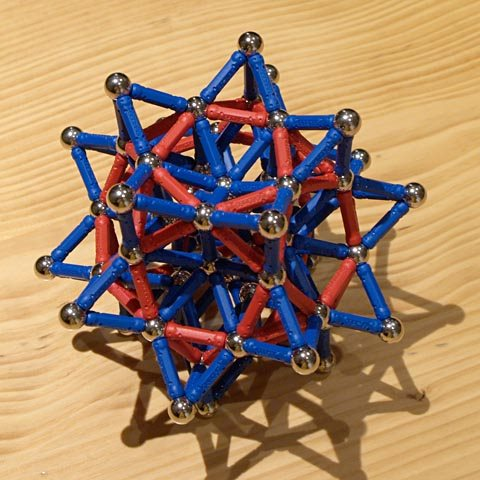Rhombic hexecontahedron built on an icosidodecahedron 242 pieces: 62 balls, 180 rods (1.38 kg)The rhombuses of the true rhombic hexecontahedron are golden rhombuses, whose angles measure about 63.43 and 116.57 degrees, while the angles in the Geomag rhombuses measure 60 and 120 degrees. Because of this, building this model has to be somewhat forced, and the resulting object is not very stable.
However, a double-scale model can be built around the single-scale one. First, we raise each pentagonal star one unit away from the center of the object. The «walls» of these raised stars are also made of rhombuses. The resulting object, which we'll call rhombic hexecontahedron to scale 1½ because it is midway towards the double-scale one, is shown in this picture:Rhombic hexecontahedron to scale 1½ 866 pieces: 194 balls, 432 rods, 240 rhombuses (4.04 kg)Unlike the single- and double-scale rhombic hexecontahedra, which can only rest on the five points of each of their 12 stars, this object can rest also on 30 sets of four vertices, and on 20 sets of three vertices:Rhombic hexecontahedron to scale 1½, on four verticesRhombic hexecontahedron to scale 1½, on three vertices
We build the points of the double-scale stars on the three-vertex sets just mentioned; the double-scale construction is finished by filling the gaps between them: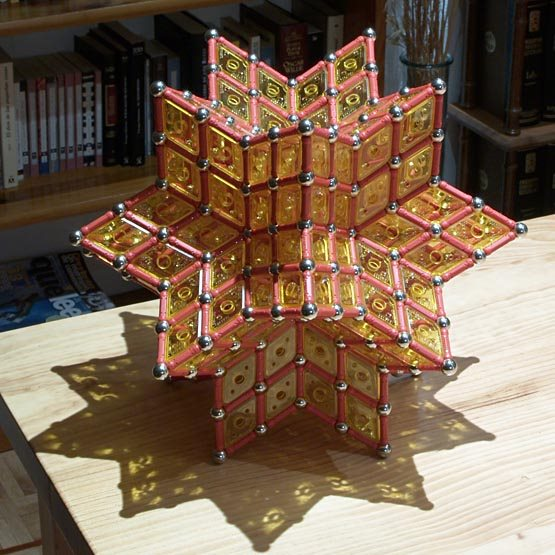Rhombic hexecontahedron to scale 2 1456 pieces: 304 balls, 732 rods, 420 rhombuses (6.66 kg)